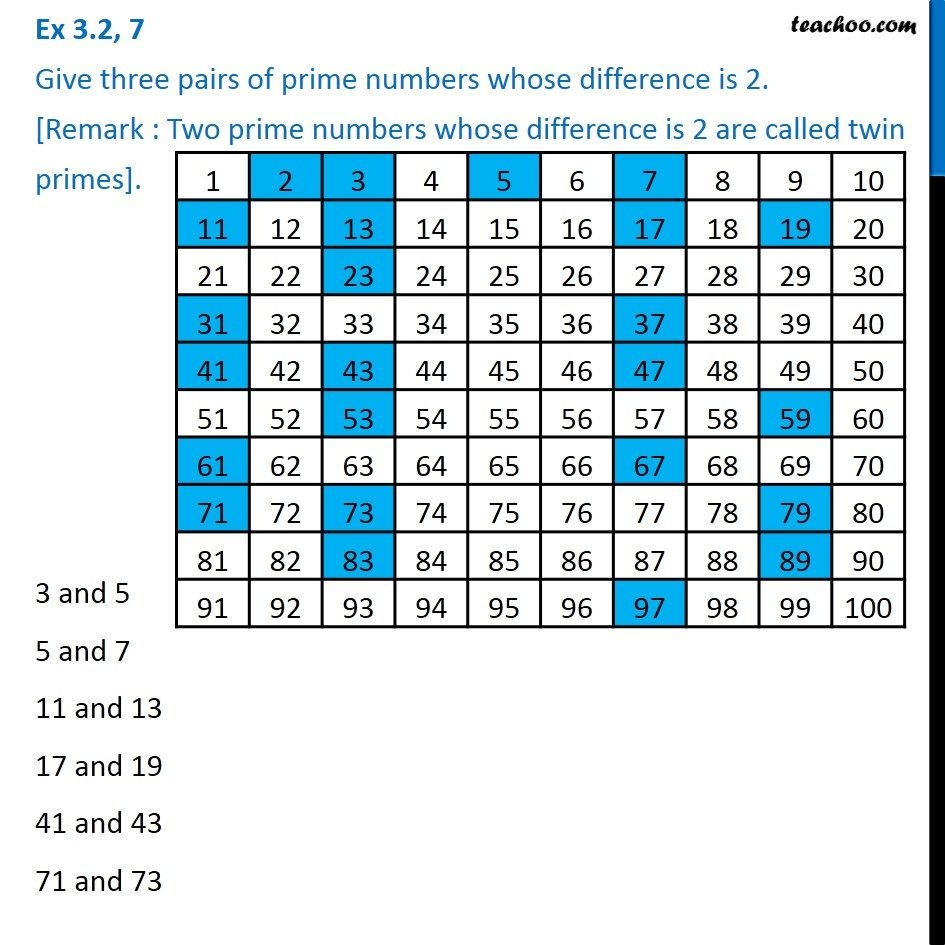1. Chapter 3 Class 6 Playing with Numbers
2. Serial order wise
3. Ex 3.2

Transcript

Ex 3.2, 7 Give three pairs of prime numbers whose difference is 2. [Remark : Two prime numbers whose difference is 2 are called twin primes]. 3 and 5 5 and 7 11 and 13 17 and 19 41 and 43 71 and 73

Ex 3.2